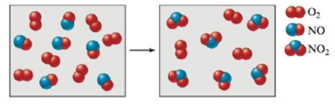# Consider the reaction between NO(g) and O 2 (g) represented below. What is the balanced equation for this reaction, and what is the limiting reactant?### Chemistry: An Atoms First Approach

2nd Edition
Steven S. Zumdahl + 1 other
Publisher: Cengage Learning
ISBN: 9781305079243

#### Solutions

Chapter
Section### Chemistry: An Atoms First Approach

2nd Edition
Steven S. Zumdahl + 1 other
Publisher: Cengage Learning
ISBN: 9781305079243
Chapter 5, Problem 115E
Textbook Problem
1 views

## Consider the reaction between NO(g) and O2(g) represented below.What is the balanced equation for this reaction, and what is the limiting reactant?

Interpretation Introduction

Interpretation: A balanced chemical equation and the limiting reactant for the given chemical reaction is to be stated.

Concept introduction: According to the law of conservation of mass, mass can neither be created nor destroyed. The mass of reactants is equal to the mass of products formed during a chemical process.

The reactant that is completely consumed during a chemical reaction is termed as the limiting reactant.

To determine: A balanced chemical equation and the limiting reactant for the given chemical reaction.

### Explanation of Solution

To determine: A balanced chemical equation for the given chemical reaction.

According to the law of conservation of mass, mass can neither be created nor destroyed. The mass of reactants is equal to the mass of products formed. Therefore a chemical equation, having lesser number of moles of an element on either side of a reaction, is balanced using appropriate numerical coefficients to satisfy the law of conservation of mass.

Adding the coefficient 2 to NO and NO2 , balances the number of atoms of nitrogen and oxygen, present on either side of the reaction. The equation thus obtained is,

2NO+O22NO2

To determine: The limiting reactant for the given chemical reaction.

Given

6mol of NO are present on the reactant side.

The stated chemical reaction is,

2NO+O22NO2

2mol of NO react with 1mol of O2

### Still sussing out bartleby?

Check out a sample textbook solution.

See a sample solution

#### The Solution to Your Study Problems

Bartleby provides explanations to thousands of textbook problems written by our experts, many with advanced degrees!

Get Started

Find more solutions based on key concepts
Vitamin C deficiency symptoms include: red spots. loose teeth. anemia. all of the above.

Nutrition: Concepts and Controversies - Standalone book (MindTap Course List)

What are the products of lipase-catalyzed hydrolysis of fats?

Introduction to General, Organic and Biochemistry

What is the monomer of a nucleic acid macromolecule?

Biology: The Dynamic Science (MindTap Course List)

What is physically exchanged during crossing over?

Human Heredity: Principles and Issues (MindTap Course List)

When the switch in Figure CQ31.8a is closed, a current is set up in the coil and the metal ring springs upward ...

Physics for Scientists and Engineers, Technology Update (No access codes included)

What happens to the energy of light when light is absorbed in seawater?

Oceanography: An Invitation To Marine Science, Loose-leaf Versin